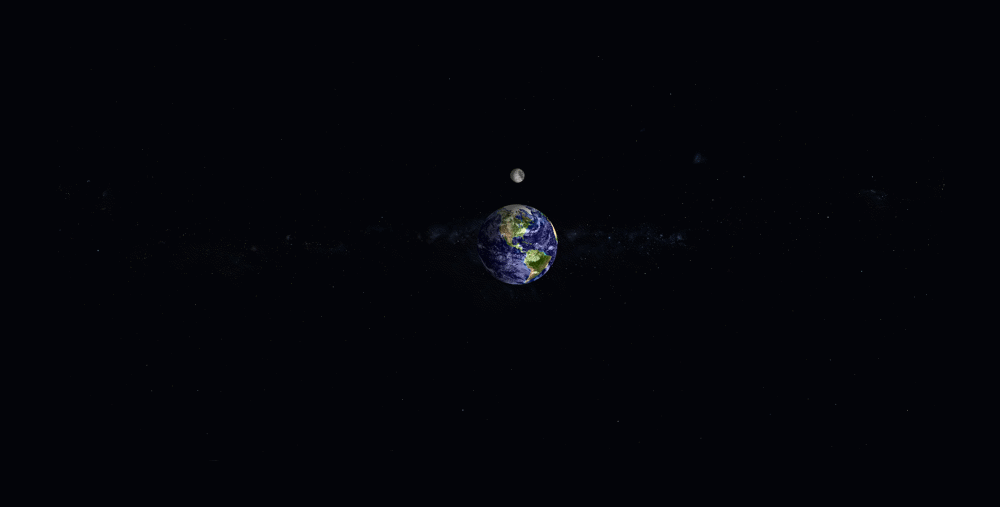# Visualizing Earth and Celestial Bodies

I'm not an expert in astronomy or space mechanics, but this entry visually caught my attention. Tamas includes a thorough documentation with plenty of examples and the details of the implementation. The planets are defined using the ellipsoid function, based on astronomical data. The planets are visualized using the surface function, and the map (texture) of the planets is defined as the CData of the surface.
I wanted to visualize the moon orbiting around Earth. I modified the function signature of planet3D to retrieve the handle of the surface object, so that I could update the location of the moon.
function planet_surface = planet3D(planet,position,gmst,reference_plane,units,transparency)
Here's the animation code. Please note that the orbit of the moon is much smaller than reality, since the objects will be too small if I use actual values.
figure
background('Milky Way');
planet3D('Earth Cloudy');
light('Position',[1,-1,0]);
% Place Moon at the origin
m = planet3D('Moon',[0 0 0]);
% Capture the X and Y coordinates of the surface object
origX = m.XData;
origY = m.YData;
% Calculate the Moon trajectory. (Value is smaller than actual, for visual
% purpose)
xd = 50000*sin(linspace(0,2*pi,100));
yd = 50000*cos(linspace(0,2*pi,100));
% Change some camera settings
camva(40)
camproj('perspective')
campos([0 -85000 30000])
camtarget('manual')
camup('manual')
for id = 1:length(xd)
m.XData = origX + xd(id);
m.YData = origY + yd(id);
drawnow
endGive it a try, and let us know what you think here or leave a comment for Tamas.
|